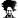# Payback period

#### bines53

##### Active Member
Hello friends,

In line 3, there is the investment (0),and a series of cash flows.(1-5).

In line 5 Cumulative Cash Flow for Payback,
The challenge, is to find the exact payback period for an investment.

The solution should be based on the data in line 5.

The emphasis is on the solution in the shortest formula and without volatile functions.

The right solution is between 2 and 3 years.

My solution next Saturday.

good luck !

David

#### Attachments

• 22.5 KB Views: 55
Last edited:

#### Hui

##### Excel Ninja
Staff member
David
It's a lot simpler than you think

=FORECAST(0,A2:F2,A5:F5)
or
=TREND(A2:F2,A5:F5,0)

•PP3321 and Thomas Kuriakose

#### bines53

##### Active Member
Hi Hui ,

Not quite accurate, real value should be 2.6876 ,

I can prove it to you in two variations,

I thought once, two formulas you mentioned, but not exact, there are two proven options,

One way, I'll give a hint, it can be done with the function LOOKUP, is another way and easier with another function, powerful implemented mostly in academia.

David

Last edited:

#### Hui

##### Excel Ninja
Staff member
Technically you are right because it is not a perfectly linear trend, however the error is 34 days, 2.6 yrs v 2.7 yrs
Nobody is going to reject a project based on that error

#### Hui

##### Excel Ninja
Staff member
=TREND(OFFSET(A2,,MATCH(0,A5:F5,1)-1,1,2),OFFSET(A5,,MATCH(0,A5:F5,1)-1,1,2),0)
=2.6876

#### Jack sparrow

##### New Member
here it is#### Attachments

• 23.5 KB Views: 51

#### bines53

##### Active Member
Hi Jack sparrow,

Very nice !

The second way, with a different function, in fact, does the same as LOOKUP, but shorter and more elegant.

David

#### Jack sparrow

##### New Member
Actually learned this formula from someone so all credit goes to him, the one who taught me without expecting anything in return

•NARAYANK991

#### bines53

##### Active Member
Hi Hui ,

https://tkljh.s3.amazonaws.com/quant/tools/excel.html.

Another version but a little different, the second argument, there is a difference when the number is negative, but is not a volatile function .

=TREND(XlOffset(A2:F2,,MATCH(0,A5:F5,1)-1,1,2),XlOffset(A5:F5,,MATCH(0,A5:F5,1)-1,1,2),0)

David

#### Chihiro

##### Excel Ninja
This?
=INTERCEPT(C2:D2,C5:D5)

#### Charles Otoghile

##### New Member
Interesting use of functions. But since it is a forecast of payback period, why insist on 100% accuracy when 99% accuracy is readily available? Still, I got something here.

#### bines53

##### Active Member
Hi Chihiro ,

It may be, can match only when you have two points, or you definitely know it's right between the two periods.

Charles,

If you place a cell E3, for example 0, zero, you will see that it is not 99%.

David

#### Chihiro

##### Excel Ninja
Well, since payback period in financial system is calculated as...
= n + (ABS(a)/b)

Where...
n = # of years before first positive cumulative cash flow
a = last negative cumulative cash flow
b = Cash flow in the year of first positive cumulative cash flow.

Therefore...
=2+(ABS(C5)/D3)
= 2 + (ABS(-65,900)/95,830) = 2.687676...

Which is equivalent of intercept between {2, 3} & {-65900, 29,930} .

#### bines53

##### Active Member
Hi Chihiro ,

Indeed, the formula is correct,=C2+(-C5/D3)

You provided we have three unknowns ,N,A ,B,
There is a function in Excel, which takes the entire range, from 0 to 5, and gives the answer,
You refer to static mode, you know the two periods and then use that function intercept.
I mean the dynamic mode, the data can vary, must constantly changes.

David

•Thomas Kuriakose and Chihiro

Ah got ya.

#### Lori

##### Active Member
David, maybe you had something like this in mind?

=PERCENTRANK(A5:F5,,20)*MAX(A2:F2)

Last edited:

#### Lori

##### Active Member
or using LOOKUP, maybe try with CSE:

=FORECAST(,IF((LOOKUP(,A5:F5,A2:F2)-A2:F2+0.5)^2<1,A2:F2),A5:F5)

#### bines53

##### Active Member
Hi Lori ,

Absolutely true !

And I am not surprised that the solution comes from you !
=PERCENTRANK(A5:F5,0,20)*MAX(A2:F2)
or excel2010 =PERCENTRANK.inc(A5:F5,0,20)*MAX(A2:F2).

On this occasion, let me ask you,
Remember the previous challenge,

=PROB(ROW(A1:A8)*{1,0,0,0},A1:A8*{1,0,0,0}+{0,9,-9,1}^99,1,ROW(A1:A8))

How do I make this formula, when it comes to columns?

To use only Line 3, I was able to do only with the function TRANSPOSE

=PERCENTRANK.INC(PROB(TRANSPOSE(COLUMN(A3:F3))*{1,0,0,0},TRANSPOSE(A3:F3)*{1,0,0,0}+{0,9,-9,1}^99,1,TRANSPOSE(COLUMN(A3:F3))),0,6)*F2

Thank you !

David

Last edited:

#### Lori

##### Active Member
David, as you say you need to use TRANSPOSE for that formula to work as it relies on the calculation going from left to right.

But in this case, i don't think you need that complicated PROB construction as the column index is available in row 2, so something like this should be ok (with CSE):

=PERCENTRANK.INC(SUMIF(A2:F2,"<="&A2:F2,A3:F3),0,6)*F2

or to allow for arbitrary time schedules (eg: 0,1,3.5,5,7):

=PERCENTILE.INC(A2:F2,PERCENTRANK.INC(SUMIF(A2:F2,"<="&A2:F2,A3:F3),0,20))

Last edited:

Hi Lori ,

You're right !

Thank you !

David

#### r1c1

Staff member
or using LOOKUP, maybe try with CSE:

=FORECAST(,IF((LOOKUP(,A5:F5,A2:F2)-A2:F2+0.5)^2<1,A2:F2),A5:F5)
An awesome formula indeed. Of course the =PERCENTRANK(A5:F5,,20)*MAX(A2:F2) one takes the cake.

But if you need a quick way to get headache, the FORECASE(,IF(...)^2) is the one to go. Trying to understand this formula without testing it in Excel makes my head hurt.#### Galen

##### New Member
or using LOOKUP, maybe try with CSE:

=FORECAST(,IF((LOOKUP(,A5:F5,A2:F2)-A2:F2+0.5)^2<1,A2:F2),A5:F5)
this one needs CSE. It has been really confusing to me when to use CSE and when not to use CSE.

#### Hui

##### Excel Ninja
Staff member
•